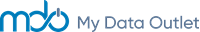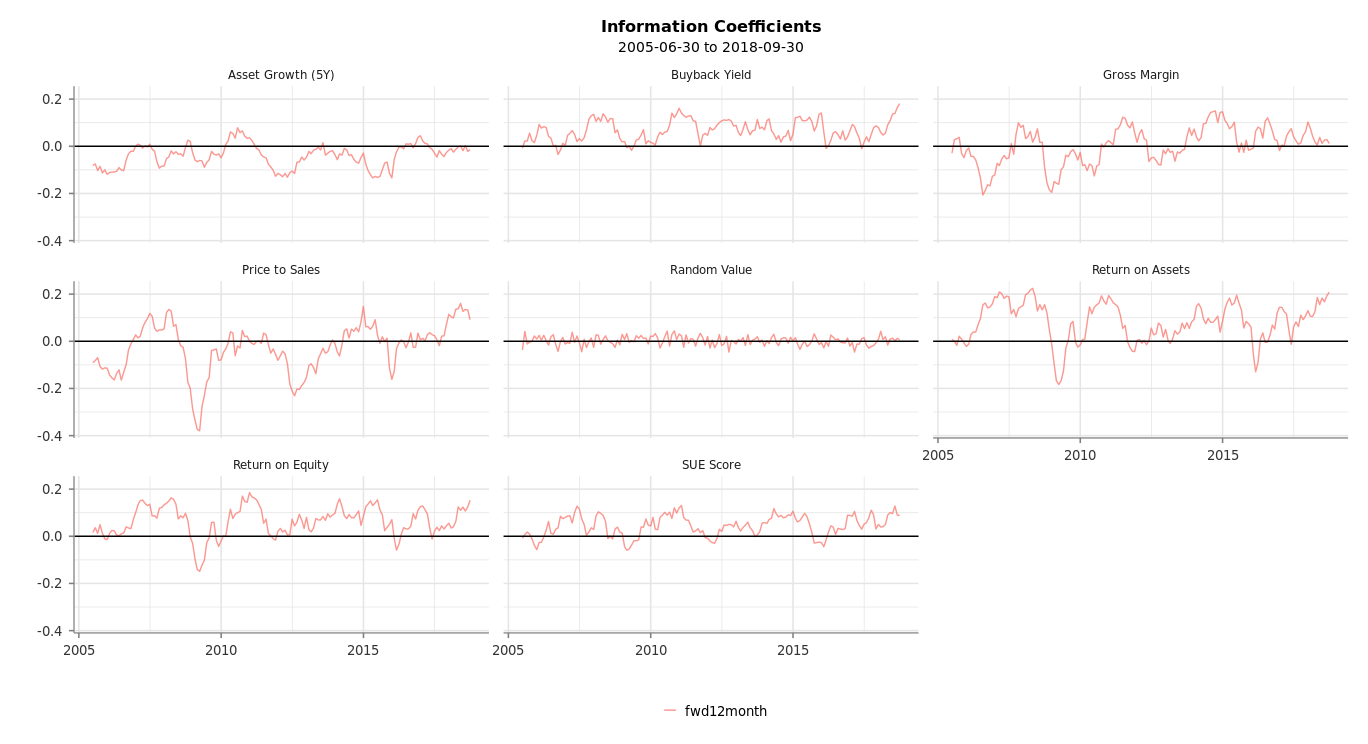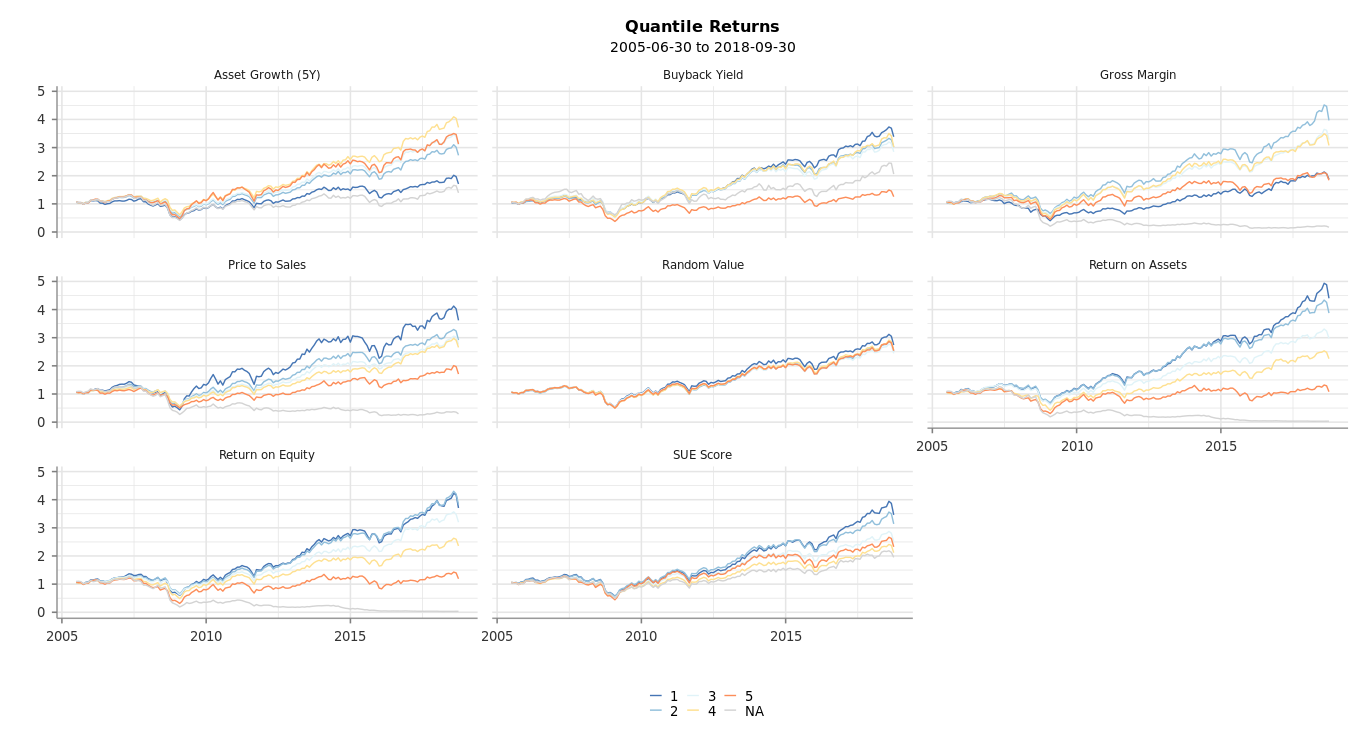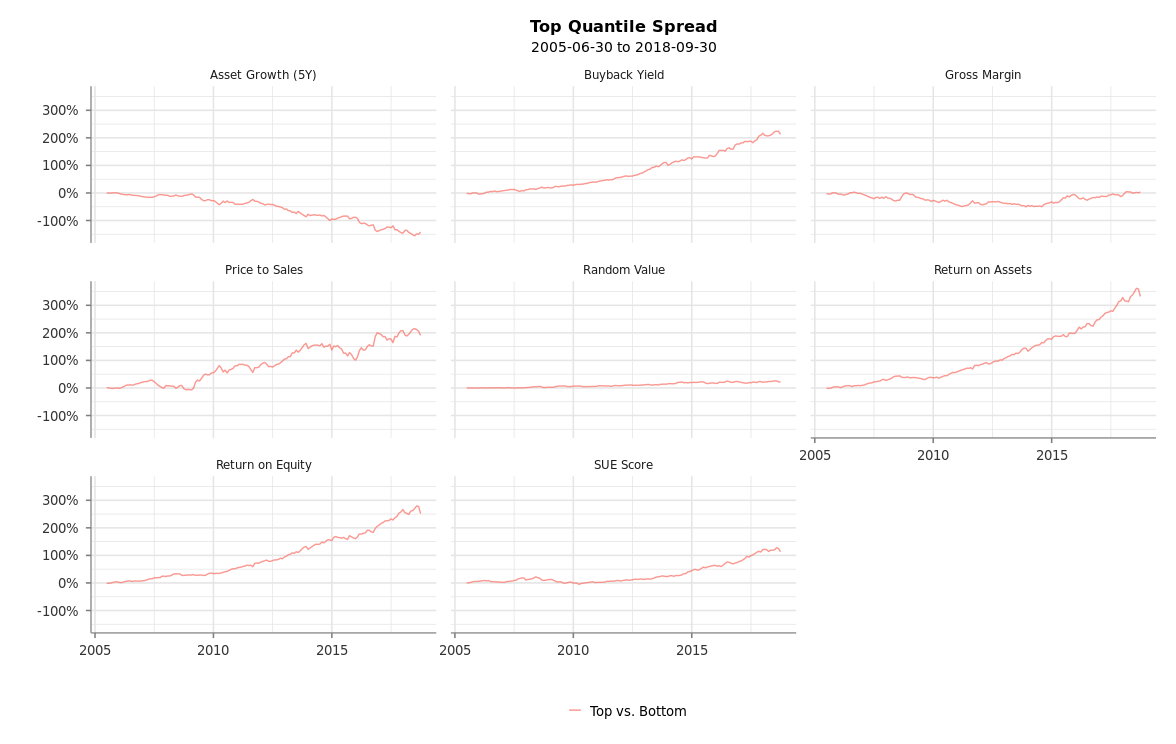Introduction

This post is intended to give readers a better understanding of the speed of the MDO data retrieval functions. The MDO Platform provides an API for users to access pricing, estimates, and fundamental data. MDO also provides functionality to easily create and test quantitative factors. We believe that the ability to run backtests in a reasonable amount of time (minutes) is critical to the research process. Every data call and calculation is tested and optimized for performance.

These examples will show some typical processing times for calculating commonly used factor formulas. For our timing, we use a universe of around 3,000 securities on month end dates from 6/30/2005 to 9/30/2018.

Summary of Results

We calculated Factor Values and Performance Statistics for around 3,000 securities on each month end date from 6/30/2005 to 9/30/2018. This totals 160 dates (> 470,000 total observations). Total backtest times were:

• Single Factor: 70 seconds
• Multi Factor: 3 minutes
• Multi Factor (custom data): 35 seconds

Single Factor Timings

For this demonstration, we will run a generic Return on Equity (ROE) factor. Let’s start by retrieving the constituents of the Russell 3000 index.

Next, we will run the QFPerformance() function on our factor, which will first calculate raw factor values. Then, QFPerformance() will calculate information coefficients and quantile peformance over various time horizons. The n = 5 argument indicates that you want to quintile the factor.

This will take around 70 seconds in total.

Multi-Factor Timings

The MDO platform also allows users to backtest multiple factors at a time, as well as models or submodels. To calculate performance for the 7 below factors (plus 1 random factor), this takes around 3 minutes in total.

Multi-Factor Timings (using custom data)

For even faster backtesting performance, you can load historical factor values into custom data tables.

In the below example, we again calculate historical factor values. We then create a custom data table and load those values. This will take around 2.5 minutes to calculate values and an additional 11 seconds to create and load the data into a SQL table.

Retrieving the raw data will take around 1 second to run, using our same historical universe.

Calculating QFPerformance() on the pre-calculated values will reduce the total time from 3 minutes to around 35 seconds. By retrieving the data from a custom data table, we can eliminate the time spent on calculating our factors on the fly. This is also useful for more granular (weekly, daily) backtesting.

Factor Performance Results

Whether we calculate data on the fly or retrieve the data from custom data, we can return the same backtest statistics and analysis to inform our decisions about these factors. The plots below show a few of the backtest statistics available in QFPerformance().Appendix

SQL Server Specs

• Operating system: Windows (Windows Server 2016 Datacenter)
• Size: Standard DS14 v2 (16 vcpus, 112 GiB memory)
• Disk: Azure Premium SSD ( 5000 IOPS limit/200 MB/s)

Factor Methodology

• ‘MDQ’ is the factor naming prefix used in the generic mdo.factors library which is provided to all clients.
• Fundamental factors use an avail of Quarterly, Semi-Annual, and Annual data.
• Fundamental factors use Quarterly TTM for Income Statement and Cash Flow items.